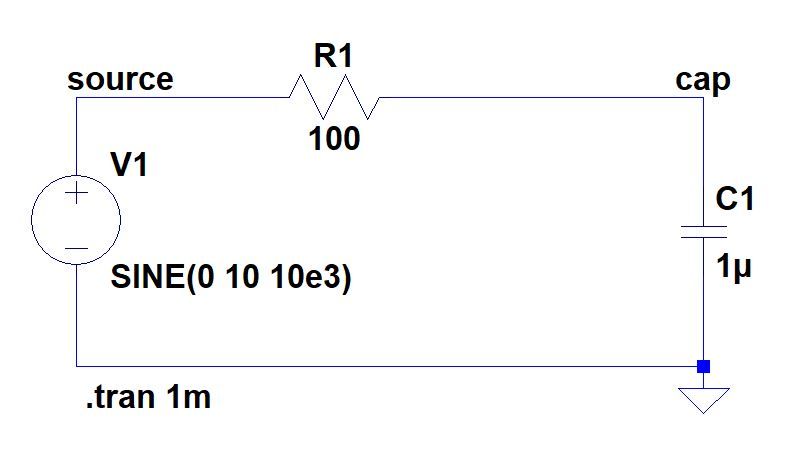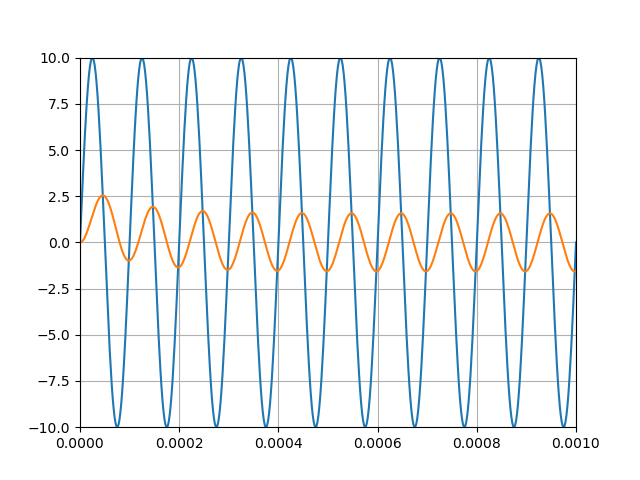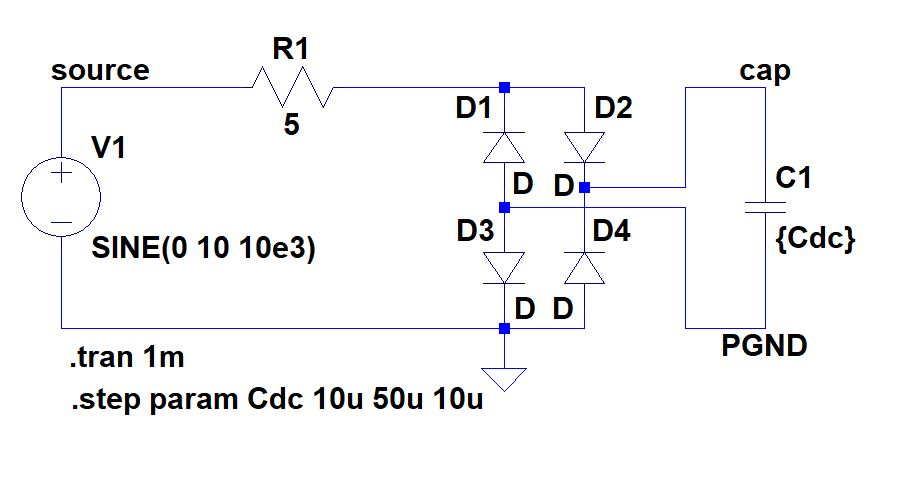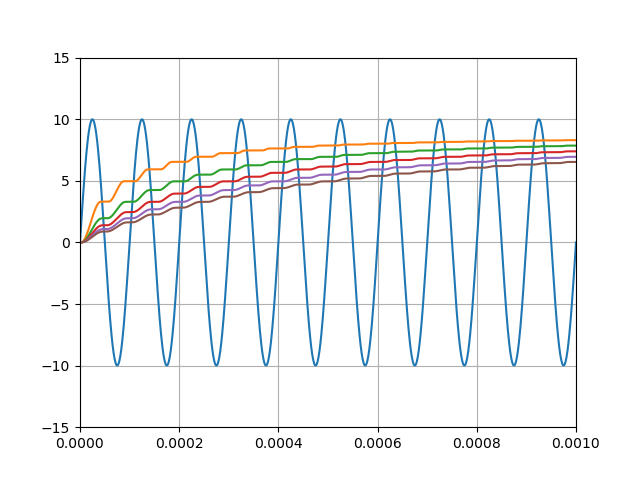DESC

# LTSpice data parsing library for python

## Installation

$pip install ltspice  ## Supported Files • encoding : UTF8 / UTF16-LE • format : Binary / Ascii • extenstion : .raw / .fft ## Usage import ltspice filepath = 'Your ltspice output file (.raw)' l = ltspice.Ltspice(filepath) l.parse() # Data loading sequence. It may take few minutes for huge file. time = l.get_time() V1 = l.get_data('V(N1)')  ## Examples ### 01 - RC Circuit #### LTSpice file (.asc)#### Python code (.py) import ltspice import matplotlib.pyplot as plt import numpy as np import os l = ltspice.Ltspice(os.path.dirname(__file__)+'\\rc.raw') # Make sure that the .raw file is located in the correct path l.parse() time = l.get_time() V_source = l.get_data('V(source)') V_cap = l.get_data('V(cap)') plt.plot(time, V_source) plt.plot(time, V_cap) plt.show()  #### Output result### 02 - Multi point simulation #### LTSpice file (.asc)#### Python code (.py) import ltspice import matplotlib.pyplot as plt import numpy as np import os l = ltspice.Ltspice(os.path.dirname(__file__)+'\\rectifier.raw') # Make sure that the .raw file is located in the correct path l.parse() time = l.get_time() V_source = l.get_data('V(source)') V_cap_max = [] plt.plot(time, V_source) for i in range(l.case_count): # Iteration in simulation cases time = l.get_time(i) # Case number starts from zero # Each case has different time point numbers V_cap = l.get_data('V(cap,pgnd)',i) V_cap_max.append(max(V_cap)) plt.plot(time, V_cap) print(V_cap_max) plt.xlim((0, 1e-3)) plt.ylim((-15, 15)) plt.grid() plt.show()  #### Output result $ [8.299080580472946, 7.855469107627869, 7.391375303268433, 6.944645524024963, 6.529755532741547]If you want to find more usage examples, please check examples folder.

## Project details

This version1.0.61.0.51.0.41.0.11.0.00.4.20.4.10.4.00.3.30.3.20.3.10.30.2.90.2.80.2.70.2.60.2.50.2.40.2.30.2.20.2.10.20.1

Uploaded source